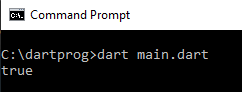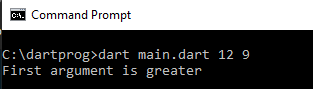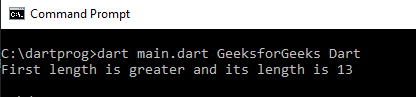Skip to content
Related Articles
Dart – Boolean
• Last Updated : 28 Feb, 2021

Dart language provides a pre-defined data type called boolean which can store two possible values, either true or false. To declare a boolean variable in Dart programming language, the keyword bool is used. Most commonly, boolean is used in decision-making statements.

The syntax for declaring a boolean value is as follows:

`Syntax: bool variable_name = true/false;`

Example 1:

The following example shows how we can assign boolean values in case of comparison of 2 predefined values.

## Dart

 `main() { ``   ``bool` `check;  ``   ``int` `val1=12;``   ``int` `val2=9;``   ` `   ``// Assigning variable check``   ``// value depending on condition``   ``check=(val1>val2);``   ``print(check);``}`

Output:Example 2:

The following example shows how we can use boolean values to check which of the two arguments passed is greater.

## Dart

 `main(List arguments) { ``   ` `   ``// Taking values of arguments``   ``// inside variables val1 and val2``   ``int` `val1=``int``.parse(arguments);``   ``int` `val2=``int``.parse(arguments);``   ``bool` `check;  ``   ` `   ``// Assigning variable check``   ``// value depending on condition``   ``check=(val1>val2); ``   ``if``(check){``       ``print(``'First argument is greater'``);``   ``}``else``{ ``       ``print(``'Second argument is greater or both are equal'``);``   ``}   ``}`

Suppose we run this dart program named main.dart using :

`dart main.dart 12 9`

Output:In the above example, as the value inside the first argument (12) is greater than the value of the second argument (9), the value inside the boolean variable check becomes true. Now, as if the condition is true the first statement is printed.

Example 3:

The following example shows how we can use boolean values to check which of the passed 2 strings is greater.

## Dart

 `main(List arguments) { ``   ``//Taking values of lengths inside variables len1 and len2``   ``int` `len1=arguments.length;``   ``int` `len2=arguments.length;``   ``bool` `check;  ``   ``//Assigning variable check value depending on condition``   ``check=(len1>len2); ``   ``if``(check){``       ``print(``'First length is greater and its length is \$len1'``);``   ``}``else``{ ``       ``print(``'Second length is greater or equal and its value is \$len2'``);``   ``}   ``}`

Suppose we run this dart program named main.dart using :

`dart main.dart GeeksforGeeks Dart`

Output:In the above example, as the length of the first string (13) is greater than the length of the second string (4), the value inside the boolean variable check becomes true. Now, as if the condition is true the first statement is printed.

My Personal Notes arrow_drop_up